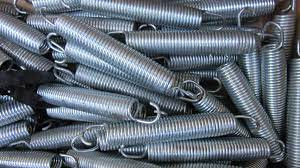-->

# Spring force definition : Here is a detailed explanation!

Spring force definition - Spring force refers to the force exerted by a spring when it is compressed or stretched from its equilibrium position. A spring is a mechanical component that is designed to store and release mechanical energy. When a spring is compressed or stretched, it exerts a force that is proportional to the distance it is displaced from its equilibrium position.This force is described by Hooke's law, which states that the force exerted by a spring is directly proportional to the displacement of the spring from its equilibrium position, and is given by the equation F = -kx, where F is the force exerted by the spring, k is the spring constant (a measure of the stiffness of the spring), and x is the displacement of the spring from its equilibrium position. The negative sign in the equation indicates that the force is opposite in direction to the displacement.

### Spring force example problems - Here are some example problems involving spring forces:

A spring with a spring constant of 100 N/m is compressed by 0.2 meters.

Solution: The formula for spring force is F = kx, where F is the force exerted by the spring, k is the spring constant, and x is the displacement of the spring from its rest position.

F = (100 N/m)(0.2 m) = 20 N

Therefore, the force exerted by the spring is 20 N.

A spring with a spring constant of 500 N/m is stretched by 0.3 meters.

Solution: Using the same formula as in the previous problem, we get:

F = (500 N/m)(0.3 m) = 150 N

Therefore, the force exerted by the spring is 150 N.

A spring with a spring constant of 50 N/m is attached to a block of mass 2 kg on a frictionless surface. The spring is compressed by 0.1 meters and then released.

What is the maximum speed of the block?

Solution: The potential energy stored in the spring when it is compressed is given by U = (1/2)kx^2, where k is the spring constant and x is the displacement of the spring from its rest position. The potential energy is converted into kinetic energy when the spring is released, so we have:

(1/2)mv^2 = (1/2)kx^2

Solving for v, we get:

v = sqrt((kx^2)/m) = sqrt((50 N/m)(0.1 m)^2/2 kg) = 1 m/s

Therefore, the maximum speed of the block is 1 m/s.

A spring with a spring constant of 100 N/m is attached to a block of mass 0.5 kg on a frictionless surface. The block is pulled back 0.2 meters from its rest position and released.

What is the maximum acceleration of the block?

Solution: The force exerted by the spring on the block is given by F = kx, where k is the spring constant and x is the displacement of the spring from its rest position. The force causes the block to accelerate, so we have:

F = ma

kx = ma

a = (k/m)x = (100 N/m)/(0.5 kg)(0.2 m) = 100 m/s^2

Therefore, the maximum acceleration of the block is 100 m/s^2.

Spring force in a sentence - The spring force is the restoring force exerted by a spring when it is stretched or compressed.

The spring force is the force exerted by a compressed or stretched spring, which is proportional to the displacement from its equilibrium position.

The spring force is the restoring force exerted by a compressed or stretched spring that tries to return it to its equilibrium position.

SeeCloseComment